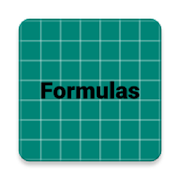# Electrical Engineering FormulasEveryone
Average value
Capacitance
Capacitive current
Capacitive reactance
Conductance
Conductivity
Current
Current divider rule
Current ratio
Electric power
Energy stored in electrostatic field of capacitance
Field intensity
Flux density
Impedance ratio
Instantaneous value of a sine wave voltage
Kirchhoff current law
Kirchhoff voltage law
Magnetomotive force
Ohm's law
Parallel circuit rules
Parallel rc circuit
Permeability
Resistance
RMS value
Self induced voltage across an inductance
Self inductance
Series circuit rules
Series rc circuits
Transformer efficiency
Turns ratio
Voltage
Voltage divider rule
Voltage ratio
Wheatstone bridge
Wye and delta conversion
Collapse

## Reviews

Review Policy

Updated
June 11, 2019
Size
3.7M
Installs
100+
Current Version
1.0
Requires Android
4.1 and up
Content Rating
Everyone
Permissions
Offered By
electronics2electrical.com